Open in App
Not now

# Hover Text and Formatting in Python-Plotly

• Last Updated : 06 Jul, 2022

Prerequisites: Python Plotly

In this article, we will explore how to Hover Text and Formatting in Python.

It is a useful approach to Hover Text and Formatting as it allows to reveal a large amount of data about complex information. One of the most deceptively-powerful features of data visualization is the ability for a viewer to quickly analyze a sufficient amount of information about data when pointing the cursor over the point label appears.

### Example 1: Default

The default setting is layout.hovermode=’closest’, wherein a single hover label appears for the point directly underneath the cursor.

## Python3

 `import` `plotly.express as px`   `df ``=` `px.data.gapminder().query(``"continent=='Oceania'"``)`   `fig ``=` `px.line(df, x``=``"year"``, y``=``"lifeExp"``, color``=``"country"``)` `fig.update_traces(mode``=``"markers+lines"``)`   `fig.show()`

Output: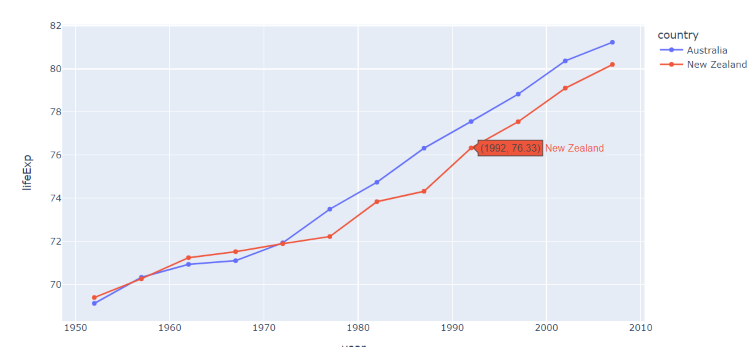### Example 2: x unified

If layout.hovermode=’x’ , a single hover label appears per trace, for points at the same x value as the cursor.

## Python3

 `import` `plotly.express as px`   `df ``=` `px.data.gapminder().query(``"continent=='Oceania'"``)`   `fig ``=` `px.line(df, x``=``"year"``, y``=``"lifeExp"``, color``=``"country"``)` `fig.update_traces(mode``=``"markers+lines"``, hovertemplate``=``None``)` `fig.update_layout(hovermode``=``"x unified"``)`   `fig.show()`

Output: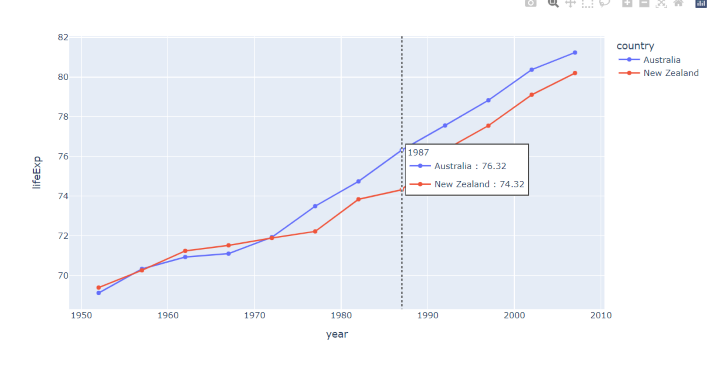### Example 3: y unified

If layout.hovermode=’y’ , a single hover label appears per trace, for points at the same y value as the cursor.

## Python3

 `import` `plotly.express as px`   `df ``=` `px.data.gapminder().query(``"continent=='Oceania'"``)`   `fig ``=` `px.line(df, x``=``"year"``, y``=``"lifeExp"``, color``=``"country"``)` `fig.update_traces(mode``=``"markers+lines"``, hovertemplate``=``None``)` `fig.update_layout(hovermode``=``"y unified"``)`   `fig.show()`

Output: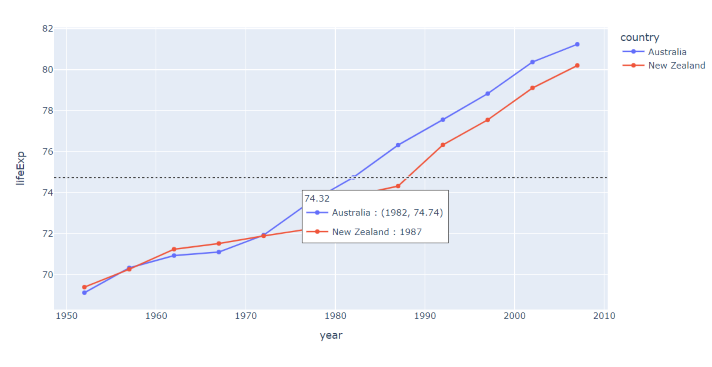## Text  Formatting

### Example 1: user_defined hovertemplate

GDP: %{x} <br>Life Expectancy: %{y}

Here, GDP and Expectancy is used as naming convention about the data whereas %{ <variable>} which allows to revel a large amount of data about complex information.

## Python3

 `import` `plotly.express as px`   `df_2007 ``=` `px.data.gapminder().query(``"year==2007"``)`   `fig ``=` `px.scatter(df_2007, x``=``"gdpPercap"``, y``=``"lifeExp"``, ` `                 ``log_x``=``True``, color``=``'continent'``)`   `fig.update_traces(hovertemplate``=``'GDP: %{x}
Life Expectancy: %{y}'``)`   `fig.show()`

Output: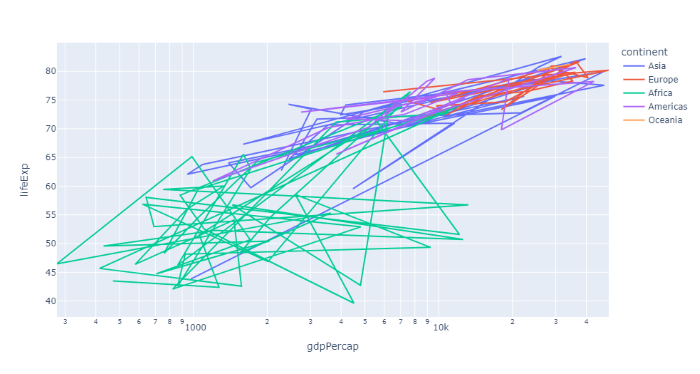### Example 2: user_defined hovertemplate

%{label}: <br>Popularity: %{percent} </br> %{text}

Here, Label, Percent, and Text is used as naming convention about the data whereas %{ <variable>} which allows to revel a large amount of data about complex information.

## Python3

 `import` `plotly.graph_objects as go`   `fig ``=` `go.Figure(go.Pie(` `    ``name ``=` `"",` `    ``values ``=` `[``2``, ``5``, ``3``, ``2.5``],` `    ``labels ``=` `[``"R"``, ``"Python"``, ``"Java Script"``, ``"Matlab"``],` `    ``text ``=` `[``"R"``, ``"Python"``, ``"JS"``, ``"Matlab"``],` `    ``hovertemplate ``=` `"%{label}:
Popularity: %{percent}
%{text}"` `))`   `fig.show()`

Output: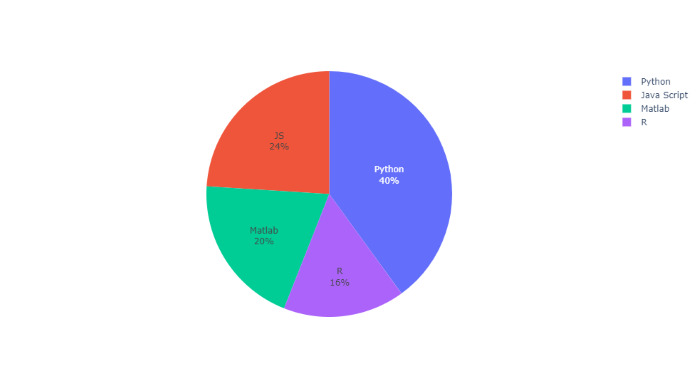My Personal Notes arrow_drop_up
Related Articles